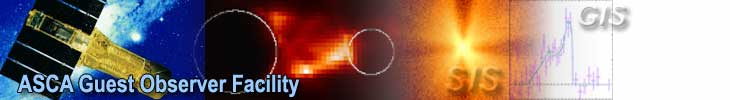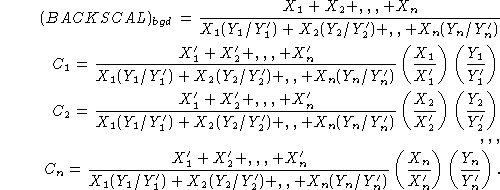Background Normalization

BACKSCAL keyword

When XSPEC subtracts the background spectrum from the source spectrum, XSPEC normalizes the background spectrum in the following way:
Background_subtracted_spectrum (cts/s) =
(SOURCE_COUNTS/SOURCE_EXPOSURE) - (BGD_COUNTS/BGD_EXPOSURE)*(SOURCE_BACKSCAL/BGD_BACKSCAL).
Here, SOURCE_BACKSCAL and BGD_BACKSCAL are the BACKSCAL keyword values in the source and background spectral files respectively, and SOURCE_EXPOSURE and BGD_EXPOSURE are the EXPOSURE keyword values.

In Xselect/Extractor, when a spectral file is created, the BACKSCAL value is defined as:

(THE DETECTOR AREA FROM WHICH THE SPECTRUM IS EXTRACTED)/(TOTAL DETECTOR AREA).

Note that it is assumed that the background is proportional to the detector area. This is not precisely true, but is considered a simple and effective approximation.

Combining background files

When source and background spectra from the two GISs or SISs are combined using mathpha, a care is needed to ensure correct amount of the background is subtracted.

Suppose s0_bg.pha is the background file for s0.pha, and s1_bg.pha is the background file for s1.pha. If s0.pha has the EXPOSURE and BACKSCAL values x0 and y0, respectively, and those of s0_bg.pha are x0' and y0', XSPEC normalizes the background by multiplying x0y0/(x0'y0') to s0_bg.pha to subtract from s0.pha. Similarly, let x1, y1, x1' and y1' have the same meanings for s1.pha and s1_bgd.pha, then x1y1/(x1'y1') times s1_bgd.pha is subtracted from s1.pha. To ensure that the right amount of background is subtracted by XSPEC for the combined spectra, calculate the following numbers:

f0 = (x0'+x1')(x0/x0')(y0/y0')/[x0(y0/y0')+x1(y1/y1')]
f1 = (x0'+x1')(x1/x1')(y1/y1')/[x0(y0/y0')+x1(y1/y1')]
out_back = (x0+x1)/[x0(y0/y0')+x1(y1/y1')]
Then
mathpha f0*s0_bg.pha+f1*s1_bg.pha F sis_bg.pha CALC 1.0
fparkey out_back sis_bg.pha+1 BACKSCAL
(use actual numerical values of f0, f1, and out_back)

will create a correct background file with EXPOSURE set to x0'+x1', i.e., the output background file will have the correct EXPOSURE time.

In the simple case of blank sky background, taken from the same areas of the detector as your source spectra (y0=y0' and y1=y1'), the above values will reduce to:

f0 = x0(x0'+x1')/[(x0+x1)x0']~1.0
f1 = x1(x0'+x1')/[(x0+x1)x1']~1.0
out_back=1.0,
since two sensors observing simultaneously will have similar exposure times (x0~x1, x0'~x1').

The above formulae can be extended to general cases when three or more non-simultaneous spectral files are combined. Suppose there are n-pairs of the source and background spectra, and X1,X2,,Xn are the EXPOSURE values for source spectra and Y1, Y2,,Yn are the BACKSCAL values. Similarly, X1',X2',,Xn' are the EXPOSURE values for the background spectra and Y1', Y2',,Yn' are the BACKSCAL values. In this case, coefficients for the background spectra to combine with and the BACKSCAL value for the combined spectrum should be the following.